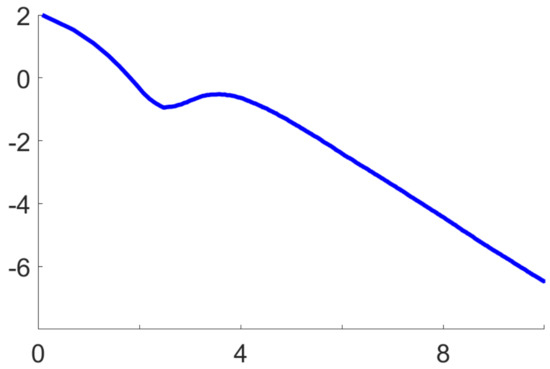Next Article in Journal
On Data-Processing and Majorization Inequalities for f-Divergences with Applications
Previous Article in Journal
Quasi-Entropies and Non-Markovianity
Open AccessArticle

# Fast, Asymptotically Efficient, Recursive Estimation in a Riemannian Manifold

byJialun Zhou 1,*,†andSalem Said 2,†1
Department of Science and Technology, University of Bordeaux, 33076 Bordeaux, France
2
IMS Laboratory, University of Bordeaux, 33076 Bordeaux, France
*
Author to whom correspondence should be addressed.
Current address: 351 Cours de la Libération, 33405 Talence Cedex, France.
Entropy 2019, 21(10), 1021; https://doi.org/10.3390/e21101021
Received: 3 September 2019 / Revised: 9 October 2019 / Accepted: 17 October 2019 / Published: 21 October 2019
Stochastic optimisation in Riemannian manifolds, especially the Riemannian stochastic gradient method, has attracted much recent attention. The present work applies stochastic optimisation to the task of recursive estimation of a statistical parameter which belongs to a Riemannian manifold. Roughly, this task amounts to stochastic minimisation of a statistical divergence function. The following problem is considered: how to obtain fast, asymptotically efficient, recursive estimates, using a Riemannian stochastic optimisation algorithm with decreasing step sizes. In solving this problem, several original results are introduced. First, without any convexity assumptions on the divergence function, we proved that, with an adequate choice of step sizes, the algorithm computes recursive estimates which achieve a fast non-asymptotic rate of convergence. Second, the asymptotic normality of these recursive estimates is proved by employing a novel linearisation technique. Third, it is proved that, when the Fisher information metric is used to guide the algorithm, these recursive estimates achieve an optimal asymptotic rate of convergence, in the sense that they become asymptotically efficient. These results, while relatively familiar in the Euclidean context, are here formulated and proved for the first time in the Riemannian context. In addition, they are illustrated with a numerical application to the recursive estimation of elliptically contoured distributions. View Full-Text
Show FiguresFigure 1

MDPI and ACS Style

Zhou, J.; Said, S. Fast, Asymptotically Efficient, Recursive Estimation in a Riemannian Manifold. Entropy 2019, 21, 1021.